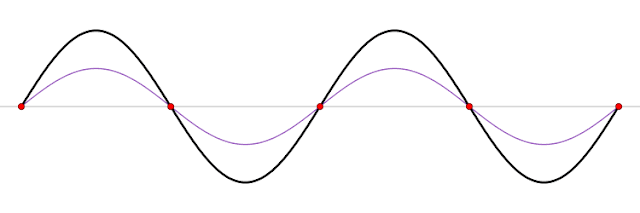## Pages

### 4.2 Principle of Superposition and Stationary waves.

One of the most important concept in the waves is the Principle of Superposition.

"The principle of superposition states that when two waves are superimposed in space, the resultant displacement at any point of space and time is the vector sum of all individual displacements at that point and time."

Example: Suppose two different waves (blue and red) starts at the same point, then the resultant displacement of propagating wave is given by the principle of superposition as shown by the wave on the right.

Stationary waves are the waves that are formed when two waves travelling in the opposite direction interfere with each other thereby producing a wave which appears to be standing still(not moving forward) and oscillating about the same position. This process of formation of the resultant wave is the clear manifestation of the applications of the superposition principle in action. The following animation shows exactly what i mean,gifcourtesy:wikipedia
In the above animation, the blue waves is moving to the right and the red wave is moving to the left, the net resultant wave as shown in the black is the stationary wave or the standing waves.

The different terminology associated with the stationary waves,

The loops shown in the above figure represents the two maximum possible amplitude for the standing waves. The blue dots where the displacement are always zero is called the nodes, the green dot are called the antinodes. The distance between the two nodes or two antinodes is always $\lambda/2$.

Click here to learn an application of the stationary waves. (To find the speed of the sound.)

Here's beautiful visualization on how to generate standing waves video,